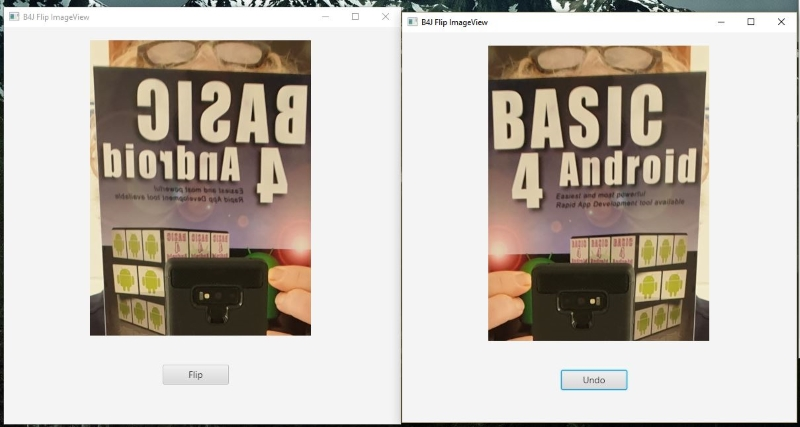# B4J Code SnippetFlip ImageView and Flip Bitmaps/Images

Was working on flipping images so I could show mirrored/reflected images correctly (and in a readable way). Tried and hoping that using the FlipCompressedBitmap method from the BitmapCreator library could help me out but I couldn't get it to work (nor understand it). Will need need to study the BitmapCreator tutorials/samples more before using it.

However, I was able to solve my problem by working directly on the ImageView itself as you can see in below screenshots.Maybe it can be useful for others too so I am sharing the code here:

B4X:
``````#Region Project Attributes
#MainFormWidth: 600
#MainFormHeight: 600
#End Region

Sub Process_Globals
Private fx As JFX
Private MainForm As Form
Private btnFlip As Button
Private imv1 As ImageView
End Sub

Sub AppStart (Form1 As Form, Args() As String)
MainForm = Form1
Dim bmp As Image = fx.LoadImage(File.DirAssets,"testmirror.jpg")
imv1.SetImage(bmp)
MainForm.Title = "B4J Flip ImageView"
MainForm.Show
End Sub

Sub btnFlip_Click
If btnFlip.Text = "Undo" Then
UndoFlipImageView(imv1)
btnFlip.Text = "Flip"
Else
FlipImageView(imv1,"Horizontal")
btnFlip.Text = "Undo"
End If
End Sub

Sub FlipImageView(imv As ImageView, Direction As String)
Dim joImv As JavaObject = imv
Dim joRotate As JavaObject
joRotate.InitializeNewInstance("javafx.scene.transform.Rotate",Null)
Dim joBounds As JavaObject = joImv.RunMethod("getBoundsInLocal",Null)
Dim bW As Double = joBounds.RunMethod("getWidth",Null)/2.0
joImv.RunMethod("setTranslateZ",Array(bW))
If Direction = "Horizontal" Then
joImv.RunMethod("setRotationAxis",Array(joRotate.GetField("Y_AXIS")))
Else if Direction = "Vertical" Then
joImv.RunMethod("setRotationAxis",Array(joRotate.GetField("X_AXIS")))
End If
joImv.RunMethod("setRotate",Array(180.00))
End Sub

Sub UndoFlipImageView(imv As ImageView)
Dim joImv As JavaObject = imv
Dim joRotate As JavaObject
joRotate.InitializeNewInstance("javafx.scene.transform.Rotate",Null)
joImv.RunMethod("setRotate",Array(0.00))
End Sub

'Return true to allow the default exceptions handler to handle the uncaught exception.
Sub Application_Error (Error As Exception, StackTrace As String) As Boolean
Return True
End Sub``````

I am also attaching it as a B4J project.

#### Attachments

• B4JFlipImageVIewDemo.zip
121.5 KB · Views: 239
Last edited:
•walt61 and Johan Schoeman

#### klaus

##### Expert
To unserstand BitmapCreator I tested some functions, the flip routine is below.
It works on the original bitmap.
B4X:
``````'flips an image
'Orientation 0 = horizontally  1 = vertically  2 = both
Public Sub Flip(Image As B4XBitmap, Orientation As Int) As B4XBitmap
Private xf, yf, x, y As Int
Private col0, col1 As ARGBColor
Private bmcImage, bmcResult As BitmapCreator

bmcImage.Initialize(Image.Width, Image.Height)
bmcImage.CopyPixelsFromBitmap(Image)
bmcResult.Initialize(Image.Width, Image.Height)

Select Orientation
Case 0
For y = 0 To Image.Height - 1
For x = 0 To Image.Width - 1
bmcImage.GetARGB(x, y, col0)
col1.a = col0.a
col1.r = col0.r
col1.g = col0.g
col1.b = col0.b
bmcResult.SetARGB(x, y, col1)
Next
Next
Case 1
For y = 0 To Image.Height - 1
For x = 0 To Image.Width - 1
xf = Image.Width - 1 - x
bmcImage.GetARGB(x, y, col0)
col1.a = col0.a
col1.r = col0.r
col1.g = col0.g
col1.b = col0.b
bmcResult.SetARGB(xf, y, col1)
Next
Next
Case 2
For y = 0 To Image.Height - 1
For x = 0 To Image.Width - 1
yf = Image.Height - 1 - y
bmcImage.GetARGB(x, y, col0)
col1.a = col0.a
col1.r = col0.r
col1.g = col0.g
col1.b = col0.b
bmcResult.SetARGB(x, yf, col1)
Next
Next
Case 3
For y = 0 To Image.Height - 1
For x = 0 To Image.Width - 1
xf = Image.Width - 1 - x
yf = Image.Height - 1 - y
bmcImage.GetARGB(x, y, col0)
col1.a = col0.a
col1.r = col0.r
col1.g = col0.g
col1.b = col0.b
bmcResult.SetARGB(xf, yf, col1)
Next
Next
End Select
Return bmcResult.Bitmap
End Sub``````

•moster67

#### moster67

##### Expert
Thanks Klaus! I actually prefer working directly with the bitmaps/images so your function is definitely the preferred way for me.
I was focusing on using the FlipCompressedBitmap method believing that was the one to use.
I have seen variations of the function you posted as well but didn't pursue that road.

Great, now we have 2 methods to obtain the same thing but with 2 different approaches. Changed the title of the thread to reflect this.

Last edited:

#### klaus

##### Expert
Just to show different ways for the horizontal flip code:
The apprentice code:
B4X:
``````Private x, y As Int
Private col0, col1 As ARGBColor
For y = 0 To Image.Height - 1
For x = 0 To Image.Width - 1
bmcImage.GetARGB(x, y, col0)
col1.a = col0.a
col1.r = col0.r
col1.g = col0.g
col1.b = col0.b
bmcResult.SetARGB(x, y, col1)
Next
Next``````
I create a new pixel color which is not necessary here so the code can be reduced to:
B4X:
``````Private x, y As Int
Private col0 As ARGBColor
For y = 0 To Image.Height - 1
For x = 0 To Image.Width - 1
bmcImage.GetARGB(x, y, col0)
bmcResult.SetARGB(x, y, col0)
Next
Next``````

And Master Erel's code:
B4X:
``````Dim c As Float = (bc.mWidth - 1) / 2
Dim argbLeft, argbRight As ARGBColor
For x = 0 To c - 0.5
For y = 0 To bc.mHeight - 1
bc.GetARGB(x, y, argbLeft)
bc.GetARGB(bc.mWidth -1 - x, y, argbRight)
bc.SetARGB(x, y,argbRight)
bc.SetARGB(bc.mWidth -1 - x, y, argbLeft)
Next
Next``````

I am working with one hand and Erel is working with two hands!
He makes half the iterations than me!

•jimmyF, DonManfred and Erel

Replies
0
Views
222
Replies
8
Views
6K
B4A Library [B4X] BitmapsAsync
Replies
5
Views
5K
Replies
92
Views
116K
Replies
41
Views
16K